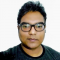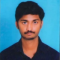## Mathematica AlternativesUpdated on 02-Nov-2023 09:04:19

59 Views

What is Mathematica? Mathematica is a mathematical computation program that has been developed by using The Wolfram Language. The app comes with a lot of features like adaptive visualization load on demand curated data, processing of images and audio, and many more. The app can be used by professionals who are in the fields of science, engineering, mathematics, and computing fields. Why Mathematica Alternatives? The app consists of a large number of functions The syntax is different in comparison to other programming languages Type commands to get answers Many shortcut notations How to choose a Mathematica Alternative? There ... Read More

## GeoGebra AlternativesUpdated on 09-Oct-2023 15:34:26

242 Views

What is GeoGebra? GeoGebra is a software that has been developed to resolve all sorts of mathematical problems for all levels of education whether primary middle, graduation, etc. The platform can resolve problems related to different subjects like geometry, algebra, statistics, graphing, and many more. Another feature of the app is the offering of one million classrooms resources for free. These are multilingual classrooms managed by a community. Price Plans of GeoGebra GeoGebra is available for free and anybody can use it to resolve different types of mathematical problems. Why GeoGebra Alternatives? There are no cons for GeoGebra How to ... Read More

## Understanding Types of MeanUpdated on 09-Aug-2023 13:39:57

211 Views

Introduction The average value of a set of data points, observations, or values is known as the Mean of the data. It is the measure of the central tendency. Mathematically, the mean is obtained by dividing the sum of values by the number of values or observations. It is also called the expected value. The mean itself is not restricted to this simple form but has different types such as the arithmetic mean, geometric mean, harmonic mean, and weighted average. It is given mathematically as, $$\mathrm{Mean=\frac{\sum x}{N}}$$ where, x = set of observations N = number of observations Different ... Read More

## Difference Between Differential and DerivativeUpdated on 30-Jun-2023 14:49:15

3K+ Views

Differential and derivative are two fundamental concepts in calculus that are often used interchangeably. While they are related, they are not the same thing. Understanding the difference between differential and derivative is important in mastering calculus and its applications. In this guide, we will explore the difference between differential and derivative and their uses in calculus. What are Differentials? Differential is one of the fundamentals divisions of calculus, along with integral calculus. It is a subfield of calculus that deals with infinitesimal change in some varying quantity. The world we live in is full of interrelated quantities that change periodically. ... Read More

## Role of Mathematics in Machine Learning workUpdated on 12-Jun-2023 16:29:46

305 Views

While attempting to understand an interdisciplinary field, for example, ML, the principal question is how much maths is vital and the degree of maths is expected to grasp these strategies. ML is about maths, which thus assists in calculating that with canning gain from data to make an exact forecast. The forecast could be as straightforward as grouping canines or felines from a given arrangement of pictures or what items to prescribe to a client in light of past buys. Thus, it is vital to understand the maths ideas driving any focal ML calculation appropriately. Along these lines, it assists ... Read More

## Difference Between Codomain and RangeUpdated on 02-Jun-2023 11:54:56

1K+ Views

In mathematics, functions play a vital role in describing and modeling various phenomena. A function is a rule that assigns a unique output value to each input value. The set of input values is called the domain, and the set of output values is called the range. However, in some cases, the range is not the same as the codomain, which can lead to confusion. In this essay, we will explore the difference between codomain and range. Codomain of a Function The “codomain” of a function or relation is a set of values that might possibly come out of it. ... Read More

## Difference Between Parallelogram and QuadrilateralUpdated on 26-Apr-2023 12:13:10

618 Views

In geometry, a quadrilateral is a polygon with four sides, while a parallelogram is a special type of quadrilateral with some specific properties that differentiate it from other quadrilaterals. What is Parallelogram? A parallelogram is a specific type of quadrilateral that has two pairs of parallel sides. In other words, the opposite sides of a parallelogram are parallel and congruent, meaning they have the same length. Parallelograms also have opposite angles that are congruent, meaning they have the same measure. Properties of Parallelogram One of the most important properties of a parallelogram is that the opposite sides are parallel. This ... Read More

## Difference Between Exponential Growth and Exponential DecayUpdated on 18-Apr-2023 16:45:10

682 Views

Exponential growth and exponential decay are two fundamental concepts in mathematics and science that describe how a quantity changes over time. Both exponential growth and decay follow a mathematical model that is characterized by a constant rate of change, but they are fundamentally different in terms of how the quantity changes over time. What is Exponential Growth? Exponential growth refers to a situation where the quantity of interest increases at an exponential rate over time. This means that the rate of increase of the quantity is proportional to the current value of the quantity. In other words, the more the ... Read More

## Difference Between ASA and AASUpdated on 17-Apr-2023 17:42:48

2K+ Views

The study of geometry is enjoyable. Sizes, distances, and angles are the primary focus of this branch of mathematics known as geometry. Shapes are the focus of geometry, a branch of mathematics. It's not hard to understand how geometry may be used to solve problems in the actual world. It finds application in a wide range of fields, including engineering, architecture, the arts, sports, and more. Today, we'll talk about a special topic in triangle geometry called congruence. But first, let's define congruence so we may use it. Whenever one figure can be superimposed over the other in such a ... Read More

## Solve the following equations and verify your answer:(i) $\frac{7x-2}{5x-1}=\frac{7x+3}{5x+4}$(ii) $(\frac{x+1}{x+2})^2=\frac{x+2}{x+4}$Updated on 13-Apr-2023 23:45:48

285 Views

Given:The given equations are:(i) $\frac{7x-2}{5x-1}=\frac{7x+3}{5x+4}$(ii) $(\frac{x+1}{x+2})^2=\frac{x+2}{x+4}$To do:We have to solve the given equations and verify the answers.Solution:To verify the answer we have to find the values of the variables and substitute them in the equation. Find the value of LHS and the value of RHS and check whether both are equal.(i) The given equation is $\frac{7x-2}{5x-1}=\frac{7x+3}{5x+4}$$\frac{7x-2}{5x-1}=\frac{7x+3}{5x+4}On cross multiplication, we get, (5x+4)(7x-2)=(7x+3)(5x-1)$$5x(7x-2)+4(7x-2)=7x(5x-1)+3(5x-1)$$35x^2-10x+28x-8=35x^2-7x+15x-3On rearranging, we get, 35x^2-35x^2+18x-8x=-3+8$$10x=5$$x=\frac{5}{10}$$x=\frac{1}{2}$Verification:LHS $=\frac{7x-2}{5x-1}$$=\frac{7(\frac{1}{2})-2}{5(\frac{1}{2})-1}$$=\frac{\frac{7}{2}-2}{\frac{5}{2}-1}$$=\frac{\frac{7-2\times2}{2}}{\frac{5-2\times1}{2}}$$=\frac{\frac{7-4}{2}}{\frac{5-2}{2}}$$=\frac{\frac{3}{2}}{\frac{3}{2}}$$=\frac{3}{2}\times\frac{2}{3}$$=1RHS =\frac{7x+3}{5x+4}$$=\frac{7(\frac{1}{2})+3}{5(\frac{1}{2})+4}$$=\frac{\frac{7}{2}+3}{\frac{5}{2}+4}$$=\frac{\frac{7+2\times3}{2}}{\frac{5+2\times4}{2}}$$=\frac{\frac{7+6}{2}}{\frac{5+8}{2}}$$=\frac{\frac{13}{2}}{\frac{13}{2}}$$=\frac{13}{2}\times\frac{2}{13}$$=1$LHS $=$ RHSHence verified.(ii) The given equation is $(\frac{x+1}{x+2})^2=\frac{x+2}{x+4}$$(\frac{x+1}{x+2})^2=\frac{x+2}{x+4}On cross multiplication, we get, (x+1)^2(x+4)=(x+2)^2(x+2)$$(x^2+2(x)(1)+1^2)(x+4)=(x^2+2(x)(2)+2^2)(x+2)$$x(x^2+2x+1)+4(x^2+2x+1)=x(x^2+4x+4)+2(x^2+4x+4)$$x^3+2x^2+x+4x^2+8x+4=x^3+4x^2+4x+2x^2+8x+8$On rearranging, we get, $x^3-x^3+6x^2-6x^2+9x-12x=8-4$$-3x=4$$x=\frac{-4}{3}$Verification:LHS $=(\frac{x+1}{x+2})^2$$=(\frac{\frac{-4}{3}+1}{\frac{-4}{3}+2})^2$$=(\frac{\frac{-4+3\times1}{3}}{\frac{-4+2\times3}{3}})^2$$=(\frac{\frac{-4+3}{3}}{\frac{-4+6}{3}})^2$$=(\frac{\frac{-1}{3}}{\frac{2}{3}})^2$$=(\frac{-1}{3})^2\times(\frac{3}{2})^2$$=\frac{1}{9}\times\frac{9}{4}$$=\frac{1}{4}RHS =\frac{x+2}{x+4}$$=\frac{\frac{-4}{3}+2}{\frac{-4}{3}+4}$$=\frac{\frac{-4+2\times3}{3}}{\frac{-4+4\times3}{3}}$$=\frac{\frac{-4+6}{3}}{\frac{-4+12}{3}}$$=\frac{\frac{2}{3}}{\frac{8}{3}}$$=\frac{2}{3}\times\frac{3}{8}$$=\frac{1}{1}\times\frac{1}{4}$$=\frac{1}{4}$LHS $=$ RHSHence verified.Read More

1 2 3 4 5 ... 31 Next